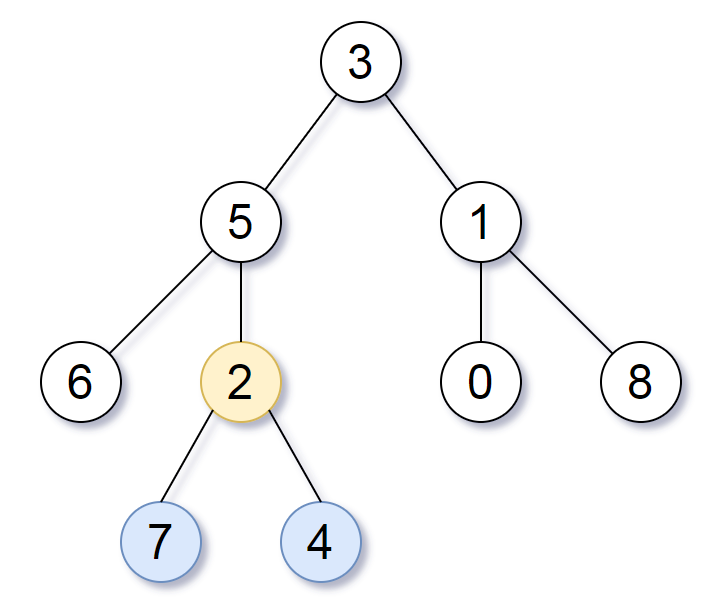# GeetCode Hub

Given the `root` of a binary tree, the depth of each node is the shortest distance to the root.

Return the smallest subtree such that it contains all the deepest nodes in the original tree.

A node is called the deepest if it has the largest depth possible among any node in the entire tree.

The subtree of a node is tree consisting of that node, plus the set of all descendants of that node.

Note: This question is the same as 1123: https://leetcode.com/problems/lowest-common-ancestor-of-deepest-leaves/

Example 1:```Input: root = [3,5,1,6,2,0,8,null,null,7,4]
Output: [2,7,4]
Explanation: We return the node with value 2, colored in yellow in the diagram.
The nodes coloured in blue are the deepest nodes of the tree.
Notice that nodes 5, 3 and 2 contain the deepest nodes in the tree but node 2 is the smallest subtree among them, so we return it.
```

Example 2:

```Input: root = 
Output: 
Explanation: The root is the deepest node in the tree.
```

Example 3:

```Input: root = [0,1,3,null,2]
Output: 
Explanation: The deepest node in the tree is 2, the valid subtrees are the subtrees of nodes 2, 1 and 0 but the subtree of node 2 is the smallest.
```

Constraints:

• The number of nodes in the tree will be in the range `[1, 500]`.
• `0 <= Node.val <= 500`
• The values of the nodes in the tree are unique.

/** * Definition for a binary tree node. * public class TreeNode { * int val; * TreeNode left; * TreeNode right; * TreeNode() {} * TreeNode(int val) { this.val = val; } * TreeNode(int val, TreeNode left, TreeNode right) { * this.val = val; * this.left = left; * this.right = right; * } * } */ class Solution { public TreeNode subtreeWithAllDeepest(TreeNode root) { } }